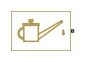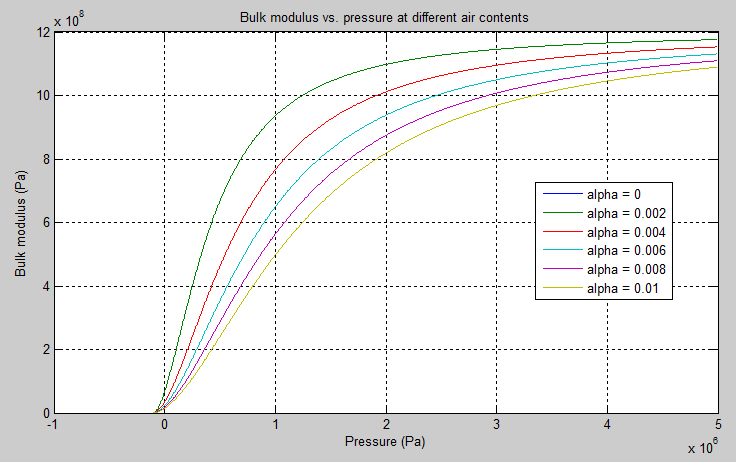# Hydraulic Fluid

(To be removed) Working fluid properties, set by selecting from list of predefined fluids

The Hydraulics (Isothermal) library will be removed in a future release. Use the Isothermal Liquid library instead. (since R2020a)

•Libraries:
Simscape / Fluids / Hydraulics (Isothermal) / Hydraulic Utilities

## Description

The Hydraulic Fluid block lets you specify the type of hydraulic fluid used in a loop of hydraulic blocks. It provides the hydraulic fluid properties, such as kinematic viscosity, density, and bulk modulus, for all the hydraulic blocks in the loop. These fluid properties are assumed to be constant during simulation time. The density is determined by the type of fluid, while kinematic viscosity additionally requires that the temperature is specified.

The bulk modulus value shown in the block dialog box is the bulk modulus of pure liquid, and is determined by the type of fluid and by the temperature. When the fluid properties are used in hydraulic blocks, such as Constant Volume Hydraulic Chamber or Variable Hydraulic Chamber, the fluid is represented as a mixture of liquid and a small amount of entrained, nondissolved gas, which is specified in the Hydraulic Fluid block as Relative amount of trapped air. The mixture bulk modulus in these blocks is determined as:

`$E={E}_{l}\frac{1+\alpha {\left(\frac{{p}_{a}}{{p}_{a}+p}\right)}^{1/n}}{1+\alpha \frac{{p}_{a}^{1/n}}{n·{\left({p}_{a}+p\right)}^{\frac{n+1}{n}}}{E}_{l}}$`

where

 `El` Pure liquid bulk modulus `p`α Atmospheric pressure α Relative gas content at atmospheric pressure, α = `VG`/`VL` `VG` Gas volume at atmospheric pressure `VL` Volume of liquid `n` Gas-specific heat ratio

The main objective of representing fluid as a mixture of liquid and gas is to introduce an approximate model of cavitation, which takes place in a chamber if pressure drops below fluid vapor saturation level. As it is seen in the graph below, the bulk modulus of a mixture decreases at $p\to {p}_{a}$, thus considerably slowing down further pressure change. At high pressure, $p>>{p}_{a}$, a small amount of nondissolved gas has practically no effect on the system behavior.Cavitation is an inherently thermodynamic process, requiring consideration of multiple-phase fluids, heat transfers, and so on, and as such cannot be accurately simulated with Simscape™ Fluids™ software. But the simplified version implemented in the block is good enough to signal if pressure falls below dangerous level, and to prevent computation failure that normally occurs at negative pressures.

The Hydraulic Fluid block offers a selection of predefined fluids. See Fluid Properties for how you can get information on the fluid properties used in the block. Once you select a fluid name, you can also specify the temperature of the fluid and the relative amount of entrained, nondissolved gas.

Each topologically distinct hydraulic loop in a diagram requires the properties of its working fluid to be specified. You can specify these properties either by selecting a predefined fluid using a Hydraulic Fluid block, or by specifying parameters of a custom fluid using a Custom Hydraulic Fluid block, which is available in the Simscape Foundation library. If no Hydraulic Fluid block or Custom Hydraulic Fluid block is attached to a loop, the hydraulic blocks in this loop use the default fluid, which is equivalent to fluid defined by a Custom Hydraulic Fluid block with the default parameter values.

### Fluid Properties

When you select a fluid type, the Fluid Properties section in the block dialog box displays these fluid property values:

• Density *kg/m^3)

• Viscosity (cSt)

• Bulk modulus (Pa)

You can also get information on the fluids and their properties through the MATLAB® command line. In the following example, the first command brings you the list of available fluids, and the second command plots the properties of a selected fluid from the list, in this case, Skydrol LD-4.

1. In the MATLAB Command Window, type:

```props = sh_stockfluidproperties ```

The system responds with a list of available fluids:

```props = skydrol_ld_4: [1x1 struct] skydrol_500_4: [1x1 struct] skydrol_5: [1x1 struct] hy_jet: [1x1 struct] f_83282: [1x1 struct] f_5606: [1x1 struct] f_87257: [1x1 struct] oil_10w: [1x1 struct] oil_30w: [1x1 struct] oil_50w: [1x1 struct] oil_sae_30: [1x1 struct] oil_sae_50: [1x1 struct] atf_dexron: [1x1 struct] iso_vg_32: [1x1 struct] gasoline: [1x1 struct] diesel_fuel: [1x1 struct] jet_fuel: [1x1 struct] water_glycol: [1x1 struct] ```
2. To plot the properties of the first fluid in the list, Skydrol LD-4, type:

` props.skydrol_ld_4.plot()`

The plot window opens:Fluid properties for the Skydrol family of hydraulic fluids were obtained from literature provided by the manufacturer, Solutia, Inc. More information is available on their website at: http://www.skydrol.com.

## Ports

### Conserving

expand all

Hydraulic conserving port that connects the block to the network. You can connect it to any point on a hydraulic connection line in a block diagram. When you connect the Hydraulic Fluid block to a connection line, the software automatically identifies the hydraulic blocks connected to the particular circuit and propagates the fluid properties to all the blocks in the circuit.

## Parameters

expand all

Hydraulic fluid type. Select one of the predefined fluids:

• `Skydrol LD-4`

• `Skydrol 500B-4`

• `Skydrol 5`

• `HyJet-4A`

• `Fluid MIL-F-83282`

• `Fluid MIL-F-5606`

• `Fluid MIL-F-87257`

• `Oil-10W`

• `Oil-30W`

• `Oil-50W`

• `Oil SAE-30`

• `Oil SAE-50`

• `Transmission fluid ATF (Dexron III)`

• `ISO VG 22 (ESSO UNIVIS N 22)`

• `ISO VG 32 (ESSO UNIVIS N 32)`

• `ISO VG 46 (ESSO UNIVIS N 46)`

• `Brake fluid DOT3`

• `Brake fluid DOT4`

• `Brake fluid DOT5`

• `Gasoline`

• `Diesel fuel`

• `Jet fuel`

• `Water-Glycol 60/40`

• `Water`

Fluid temperature, in degrees C.

Amount of entrained, nondissolved gas in the fluid. The amount is specified as the ratio of gas volume at normal conditions to the fluid volume in the chamber. Therefore, the parameter value must be less than 1. In practice, the relative amount of trapped air is always greater than 0. If set to 0, ideal fluid is assumed.

Proportionality coefficient that you can use to adjust fluid viscosity, if needed. Specify a value between 0.5 and 1.5.

Determines how the block handles the out-of-range assertion during simulation:

• `Error` — If the pressure falls below absolute zero, the simulation stops and you get an error message.

• `Warning` — If the pressure falls below absolute zero, you get a warning but the simulation continues. Use this option when modeling systems where cavitation can occur in extreme cases.

## Version History

Introduced in R2006a

expand all